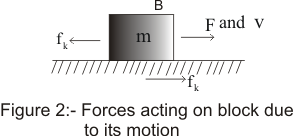# Kinetic Friction

## (3) Kinetic or dynamic friction

• We already know that static frictional force exists between two surfaces in contact before there is relative motion between two surfaces
• When applied force becomes greater then limiting friction force,then motion of the body starts and kinetic friction comes into existence
• Thus when bodies in contact moves relative to each other then friction developed between them is called kinetic or dynamic friction
• Consider the figure below in which a block of mass m is kept over the surface S• Initially the block is at rest,now we push the block on the surface such that it begins to move or start sliding on the surface
• When the block is sliding over the surface each body exerts a frictional force on the other side parallel to the surface in contact
• The Force of friction acting on the block B due to surface S is along the direction opposite to the motion of B with respect to S.Thus force of kinetic friction opposes the relative motion between the bodies in motion
• The frictional force acting on the block is along the direction towards the left and an equal force acts on the surface S directed towards the rights
• Thus we can say that sliding friction on a body B against surface S is opposite to the velocity of the body B with respect to S
• Force of kinetic friction is denoted by fk and its magnitude is always less then the magnitude of limiting friction i.e maximum static friction
i.e fk < fms
• kinetic friction force is proportional to the normal contact force between the surfaces i.e
fk is proportional to N
=> fkkN
Where N =Normal contact force
μk is the proportionality constant known as coefficient of kinetic friction and its value depends on the nature of the two surfaces in contact
• fk < fms, this inequality shows that force required to start the motion is greater then the force required to maintain the further motion of the body